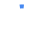ExpiredFractions math Pro

\$0.99 FREE

This calculator is the pro version of 'Fractions Math', and completely without advertisement.

This math app is a fractions calculator with various functions:

- add, subtract, multiply and divide fractions.
- convert fractions in decimals,
- simplify fractions
- compare fractions
- find the lowest common denominator
- fraction trainer, to learn fraction calculations

Thanks for voting !!
 100% 0%Subscribe To Get All Offers In Your Inbox Every Day